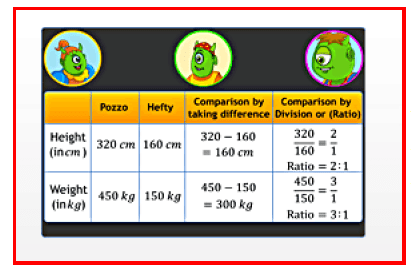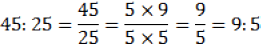Courses

# Chapter Notes - Ratios and Proportions Class 6 Notes | EduRev

## Class 6: Chapter Notes - Ratios and Proportions Class 6 Notes | EduRev

The document Chapter Notes - Ratios and Proportions Class 6 Notes | EduRev is a part of the Class 6 Course Mathematics (Maths) Class 6.
All you need of Class 6 at this link: Class 6

12. Ratios and Proportions

Ratios:Usually, the comparison of quantities of the same type can be made by the method of difference between the quantities.

Usually, the comparison of quantities of the same type can be made by the method of difference between the quantities. However, a more meaningful comparison between the quantities can be made by using division, i.e. by verifying how many times one quantity is into the other quantity. This method is known as comparison by ratio.

For example, Keertana's weight is 20 kg and her father's weight is 80 kg. So we can say that Keertana's father's weight and Keertana's weight are in the ratio 20:80.

To calculate ratio, the two quantities have to be measured using the same unit. If not, they should be converted to the same unit before ratio is taken. The same ratio can occur in different situations. For example, the ratio 4:5 is different from 5:4.

Thus, the order in which the quantities are taken into consideration to express their ratio is important.

A ratio can be treated as a fraction.

For example, 5:6 can be treated as 5/6. Two ratios are said to be equivalent if the fractions corresponding to them are equivalent.

To calculate equivalent ratio, convert the ratio into a fraction, and then multiply or divide the numerator and the denominator by the same number. Ex:4:5 is equivalent to 8:10 or 12:15 and so on. A ratio can be expressed in its lowest form. For example, the ratio 45:25 in its lowest form can be written as follows:Thus, the lowest form of 45:25 is 9:5.

Page 53

Proportions:If the ratios between Quantity A and Quantity B is equal to the ratio between Quantity C and Quantity D,

If the ratios between Quantity A and Quantity B is equal to the ratio between Quantity C and Quantity D, then the four quantities A, B, C and D, are said to be in proportion. Proportion is denoted by the signs '?' or '='. Thus, the quantities 4, 16, 5 and 20 can be written as 4:16?5:20 or 4:16=5:20 The order of the terms in a proportion carries value. The quantities 4, 16, 5 and 20 are in proportion, whereas 4, 20, 5 and 16 are not in proportion. In the proportion a:b?c:d, the quantities a and d are the extreme terms, and b and c are the middle terms. The method of calculating the value of one unit and using this value to calculate the value of the required number of units is called the unitary method.

For example, suppose the cost of 8 bags is Rs. 240. Now, to find the cost of 6 bags,
using the unitary method, we first find out the cost of one bag. Cost of one bag =240/8 = Rs. 30

Now, the cost of 6 bags =6 × Rs.30=180 Hence, the cost of 6 bags is Rs. 180.

The document Chapter Notes - Ratios and Proportions Class 6 Notes | EduRev is a part of the Class 6 Course Mathematics (Maths) Class 6.
All you need of Class 6 at this link: Class 6Use Code STAYHOME200 and get INR 200 additional OFF Use Coupon Code
All Tests, Videos & Notes of Class 6: Class 6

### Top Courses for Class 6## Mathematics (Maths) Class 6

185 videos|229 docs|43 tests

### Top Courses for Class 6Track your progress, build streaks, highlight & save important lessons and more!

,

,

,

,

,

,

,

,

,

,

,

,

,

,

,

,

,

,

,

,

,

;